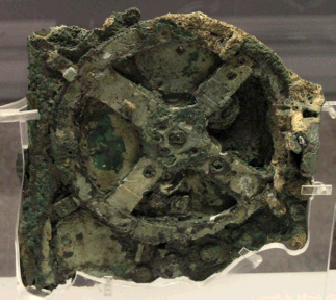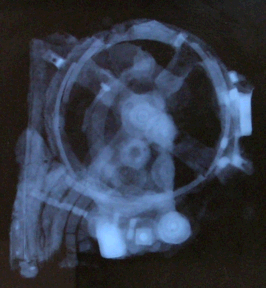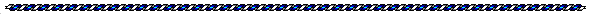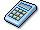The Antikythera Mechanism   Converting To Ancient Gematria Numbers  By Jerry Iuliano  Compiled by Joseph E. Mason

Introduction
(From Joseph E. Mason)

Jerry Iuliano has discovered some amazing number relationships in the ancient artifact known as the Antikythera Mechanism. The object was found after the discovery in 1901 of an ancient shipwreck near the Island of Antikythera, which is near Crete in the Mediterranean Sea. The ship dates back to the first century BC. The mechanism, dated to around the end of the second century BC, was found to be an extremely complex device, with many gears and axles. Nothing as complex had been found to exist until at least a thousand years later.Image Credit: The American Philosophical Society, 1974

The mechanism may be the first known computing device. Its function was apparently to show the relationships of the Sun, the Moon, and some of the planets, in relation to the Zodiac, displayed on three dials, one on the front, and two on the back. Also, the rising and setting of specific stars were thought to be indicated by the front dial.

New information came to light recently, reported in the science journal Nature on 30 November 2006.

The mechanism predicted lunar and solar eclipses on the basis of Babylonian arithmetic-progression cycles. The inscriptions support suggestions of mechanical display of planetary positions now lost.

Orrery maker, John Gleave, constructed a working replica of the Antikythera Mechanism:Image Credit: A Reconstruction of the Antikythera MechanismThe Antikythera Mechanism
Converting To Ancient Gematria Numbers
By Jerry Iuliano

 Results 1 - 100 of about 1,170,000 for inches in one meter. (0.17 seconds)  inches: http://www.answers.com/inches&r=67one meter = 39.3700787 inches

. . . at the last website is the complete schematic breakdown of the device, shown in five gear colors, blue, yellow-sun gear, green, orange, purple and dark green. Thirty gear numbers are shown, each having one of the above colors. The key number constant used in all thirty gears is the modern metric to english conversion constant . . . ( 39.3700787 inches = 1 meter ) . . . rewritten to this form:

 39.3700787 / ( 10 ^ 3 )  = m = 1/25.4

Image Credit: The Antikythera Mechanism Research Project (copyright)
(The graphic above is resized to show the significant details)

. . . all thirty gears are linked and can be turned all at once by the rotation of any one gear in the system. I chose the blue (50) gear as a starting point and turned this gear exactly one-revolution or 360 degrees. The gematric formula for this angle is the sacred number 72,  times one half the metric standard 254
( 254/2 = 127 ):

 72 * 127 * m =  360 degrees

. . . this angle is transferred to the second blue(50) gear because of its being self similar to the first blue(50) gear. This angle is also  transferred to the blue(32) gear because of a shared axle. The next step is the blue(32) gear's link to gear, blue(127). This link is the famous sacred Alautun time cycle number . . . 2304 . . . from Aztec and Mayan culture.

 blue(127) =  2304 * m    =     32 / 127  * 360 = 90.70866132 degrees blue(24)  =  2304 * m    =     32 / 127  * 360 = 90.70866132 degrees

. . . that is when you turn the starter blue(50) gear 360 degrees, the blue(127) gear will turn 90.70866132 degrees. The blue(24) gear shares an axle with the blue(127) gear and thus shares the rotation of the blue(127) gear. Blue(24) gear then transfers this rotation to the blue(48) gear. This angle of rotation is the gematric number known as the Egyptian foot number . . . 1152 . . .

 blue(38) =  1152 * m = 32/127*24/48*360 = 45.35433066 degrees blue(48) =  1152 * m = 32/127*24/48*360 = 45.35433066 degrees

. . . blue(38) shares an axle with blue(48) and thus transfers this angle of rotation to the so-called Sun gear . . . yellow(64) . . . The number 19 appears here not as years of the Metonic cycle but as DAYS!! on the edge of gear wheels:

 Sun gear  =   36 * 19 * m = 32/127*24/48*38/64*360 = 26.92913383 degrees

. . . all of the green gears share the same angle of rotation as the Sun gear. The orange gears appear when the second gear with 38 cogs appears as orange(38). It has the same angle of rotation as the blue(38) gear:

 orange(38) = 1152  *  m = 32/127*24/48*360 =  45.3543306 degrees orange(53) = 1152  *  m = 32/127*24/48*360 =  45.3543306 degrees

. . . orange(53) shares an axle with orange(38) and thus transfers this angle of rotation to orange(96)

 orange(96) =  25 + m  =  53*12 * m = 32/127*24/48*53/96*360 = 25.0393700787 degrees

. . . orange(15) and orange(27) gears share axles with orange(96) and thus transfers angular rotation to the large gear purple(223):

 purple(223) = 53*12*27*m/223 = 36*m+360/223 = 32/127*24/48*53/96*27/223*360 degrees

. . . purple(53) edges with purple(223) thus picking up an angular rotation of:

 purple(53) = 54 * 6 * m = 32/127*24/48*27/96*360 = 12.7559055 degrees purple(30) = 54 * 6 * m = 32/127*24/48*27/96*360 = 12.7559055 degrees

. . . purple(30) shares an axle with purple(53) and thus the same rotation. Purple(30) edges with purple(54) resulting in an angle turn of 7.086614166 degrees for purple(54):

 purple(54) = 180 * m  = 7.086614166 degrees

. . . three other gears share this angle of rotation and thus the same axle, purple(20), dk green(53), dk green(15). dk green(15) transfers the angle to gear dk green(60) and gear dk green(12) which shares an axle with dk green(60):

 dk green(60) = 180 / 4  * m  =  32/127*24/48*15/96*15/60*360 = 1.771653542 degrees dk green(12) = 180 / 4  * m  =  32/127*24/48*15/96*15/60*360 = 1.771653542 degrees

. . . dk green(12) transfers the angle of rotation to the last gear in the chain, dk green(60)

 dk green(60) = 9 * m = 32/127*24/48*15/96*15/60*12/60*360 = .354330708 degrees

. . . showing the angles of rotation in spreadsheet form: cl = clockwise, ccl counterclockwise

 GEAR GEMATRIA GEAR RATIO ROTATION ANGLE blue(50) 72 * 127 * m 1 360 ccl blue(50) 72 * 127 * m 1 360 cl blue(32) 72 * 127 * m 1 360 cl blue(127) 2304   *   m 32/127*360 90.70866132 ccl blue(24) 2304   *   m 32/127*360 90.70855132 ccl blue(48) 1152  *   m 32/127*24/48*360 45.35433066 cl blue(38) 1152  *   m 32/127*24/48*360 45.35433066 cl sun gear(64) 36 * 19 * m 32/127*24/48*38/64*360 26.92913383 cl green(32) 36 * 19 * m 32/127*24/48*38/64*360 26.92913383 cl green(32) 36 * 19 * m 32/127*24/48*38/64*360 26.92913383 ccl green(50) 36 * 19 * m 32/127*24/48*38/64*360 26.92913383 ccl green(50) 36 * 19 * m 32/127*24/48*38/64*360 26.92913383 cl orange(38) 1152 * m 32/127*24/48*360 45.35433066 ccl orange(53) 1152 * m 32/127*24/48*360 45.35433066 ccl orange(96) 53*12 * m = 25+m 32/127*24/48*53/96*360 25.0393700787 cl orange(15) 53*12 * m = 25+m 32/127*24/48*53/96*360 25.0393700787 cl orange(27) 53*12 * m = 25+m 32/127*24/48*53/96*360 25.0393700787 cl purple(223) 53*12*27*m/223 32/127*24/48*53/96*27/223*360 3.031672609 ccl purple(53) 54 * 6 * m 32/127*24/48*27/96*360 12.7559055 cl purple(30) 54 * 6 * m 32/127*24/48*27/96*360 12.7559055 cl purple(54) 180 *  m 32/127*24/48*27/96*30/54*360 7.086614166 ccl purple(20) 180 *  m 32/127*24/48*27/96*30/54*360 7.086614166 ccl purple(60) 60 *  m 32/127*24/48*27/96*30/54*20/60*360 2.362204722 cl purple(15) 60 *  m 32/127*24/48*27/96*30/54*20/60*360 2.362204722 cl purple(60) 15 *  m 32/127*24/48*27/96*30/54*20/60*15/60*360 .59055118 ccl dk green(53) 180 * m 32/127*24/48*27/96*30/54*360 7.086614166 ccl dk green(15) 180 * m 32/127*24/48*27/96*30/54*360 7.086614166 ccl dk green(60) 180/4 * m 32/127*24/48*15/96*15/60*360 1.771653542 cl dk green(12) 180/4 * m 32/127*24/48*15/96*15/60*360 1.771653542 cl dk green(60) 9 * m 32/127*24/48*15/96*15/60*12/60*36 .354330708 ccl

. . . how does  this data relate to the earth-moon interaction? One can observe that the last gear in the chain of 360 degree rotation, blue(32) . . . if one makes this gear analogous to the moon lunar cycle . . . 29.530588 days in one orbit around earth, then blue (32) and the lunar cycle share an axle that rotates 360 degrees. Transferring this 360 degree rotation of the lunar cycle to the earth cycle around the sun . . . 365.246743 days in one tropical year,  on this earth cycle axle, place the 5 sets of 47 (235) markings of the exterior dials of the device on the earth axis of rotation and multiply by the metric m:

 29.530588 / 365.2467463 * 5 * 47 *m * 36 = 26.92913386 degrees = 19 * 36 * m = sun gear(64) 29.530588 / 365.2467463 * 235 / 254 = 26.92913386 degrees = 19 * 36 * m   = sun gear(64)

. . . this is exactly the angle turned by the sun gear when the blue(50) gear is rotated once!! Checking the number constants I wanted to see if the number 37 is anywhere in the angles of rotation or in the gear numbers. Strangely it sits at the heart of the Earth/Moon link. When the lunar cycle 29.530588 days is rotated once (360 degrees) on the Earth cycle . . . 365.246743 days . . . an angle of rotation is generated on the Earth cycle rim:

 29.530588 / 365.246743 * 360 = degrees of rotation of Earth orbit disc = 29.10638332

. . . 37 derives this angle through the 47 markings on the outside dials of the device:

 (( 37 ^ 2 ) - 1 ) / 47  = 29.10638332 degrees

. . . which means the 37 form can generate the sun gear angle for all of the green gears:

 (( 37 ^ 2 ) - 1 ) / 47  * 235  / 254 = 26.92913386 degrees = 36 * 19 * m

. . . are the other planets represented on the Antikythera mechanism? The set up is related to the gematric numbers . . . 1152, 2304, 180, 36 . . . etc . . . multiplied by the modern metric to inch conversion constant . . . m = 39.3700787 / ( 10 ^ 3 ) . . . and the gear numbers are representing the symbolic and correct gear ratios of the Sun, Moon, and Earth associations to their respective orbits around the sun measured in Earth days. The critical measure here is the association of the gear cogs in the wheels to planetary orbits measured in Earth days. Other than the moon, the brightest planets in the sky and their Earth day measured tropical year in one orbit are as follows: (Wikipedia)

 VENUS = 224.70069 days . . . used in formula 224.6842679 MARS = 686.96 days . . . used in formula 686.9439531 JUPITER = 4333.2867 days MERCURY = 87.96955 days . . . used in formula 87.96497629 EARTH = 365.2467463 days

. . . it was discovered that the Venus to Earth ratio multiplied by the Sun(64) gear was the source of the metric value m:

 VENUS / EARTH * SUN(64) / ( 10 ^ 3 ) = m

. . . if the Venus gear 224.6842679 (imaginary) is rotated 360 degrees alongside the Earth gear 365.246742 (imaginary), then this angle of rotation is the Sun gear's 64 (real) rotation since it is attached to the same axle as the Earth gear. The Sun gear's rotation is transferred to an imaginary gear with a circumference of ( 10^3 ). This value is m and it is a percentage, NOT an angle rotation. To find the angle rotation of the 10^3 gear multiply by 360 or m * 360 degrees equals the angle turn of the scalar gear 10^3 when the Venus orbit(gear) is rotated one revolution, 360 degrees. The only thing real in the equation is the Sun gear(64). This gear is key in the Antikythera device as to an explanation of the Mars, Jupiter, and Mercury orbits. How this formula was discovered, I can only comment that it "appeared" after working with the Sun gear's ratios and planetary orbits. This discovery goes to the heart of how immensely amazing the mechanical device is and how, whoever built it had a knowledge of astronomy and timekeeping that parallels modern measurement. The first planet, Mercury, is attached to the device's ( 224 ) gear that sits atop the Sun gear(64) through the metric constant m:

 MERCURY / 224 + .001 = 10 * m

. . . the tetrakys 10 is key to the understanding of the remaining planetary orbits of Mars and Jupiter. If you rotate the Mars gear ( 686.9439531 ) exactly one revolution, 360 degrees, and transfer this rotation to the Jupiter gear ( 4333.2867 ) which is attached to the same axle as the Sun gear (64), and let the Sun gear(64) transfer this angle of rotation to the Earth gear ( 365.246742 ), the rotation placed upon the Earth gear is exactly 10 degrees!! Now this is incredible, the entire system of planetary gearing will create an exact logarithmic integer of rotation when the Sun gear is exactly 64. Also, the deepest meaning of the Alautun number 2304 from the device is shown as a three planet ratio involving Jupiter, Mars and the Earth!!

 ALAUTUN (2304) = JUPITER * EARTH / MARS SUMERIAN (36) = JUPITER * EARTH / MARS / SUN(64) MARS / JUPITER * SUN (64) / EARTH * 360 = 10 degrees . . . exact

. . . that is during one rotation of the Mars orbit frequency, when transferred to the gear chain above, the earth will rotate exactly 1/36th the part of its circumferential orbit about the sun. There are several gear trains in the Antikythera device that could use this information to perform this task of translating 1/36th Earth orbit change to a gear ratio. Here are some of the gears which are all multiples of 36 using 18 as a factor:

 all green gears.......................36 * 19 * m purple gears (54) and (20)..........180 * m dk green gears (53) and (15) .....180 * m

. . . the interesting thing about all of these forms is that they are used in Mayan mythology and in their calendar systems as shown below at this web site:

. . . this is very important to the explanation of the Antikythera device because both the 1152 and 2304 units are Mayan calendar constants measured in DAYS!! -- the same as the Antikythera mechanism!!

"The unit of the Mayan calendar was the day, or kin. Following the chronological vigesmal system, 20 kins made up a uinal( or month ), 18 uinals ( the third position of the chronological system ) formed a tun, ( or a year of 360 days ):

 20 tuns = 1 katun or cycle of 7200 days ( Antikythera device ) 20 katuns = 1 baktun or cycle of 144000 days 20 baktuns =1 pictun or cycle of 2880000 days 20 pictuns = 1 calabtun or cycle of 57600000 days 20 calabtun = 1 kinchiltin = a cycle of 1152000000 days ( Antikythera device ) 20 kinchiltin = 1 alautun = a cycle of 23040000000 days ( Antikythera device )

. . . these nine periods of time, from the kin until the alautun, each with it's glyph were used by the Mayan to date inscriptions in their measurements . . . "

_____________

Investigating all planets, including the invisible ones, Uranus and Neptune, led to the most accurate measurement of the planetary orbits yet, when placed into a gear train and compared to the original revolutions of the Earth - Lunar relationship. The Earth-Moon relationship uses the number 19 and the Metonic constant ( 235 ) and the Saros derivative ( 254 ) lunar phase to match with the device's Sun gear(64):

 Moon / Earth * 235 / 254 * 360 = angle turn of Sun gear(64) when Moon gear(32) rotated once

. . . incredibly after investigation, ALL eight planets of the solar system were found to use the Metonic constant ( 235 ) as the main analogy to actual planetary orbits when measured in orbital Earth days. Strange that the exact number for the constant for such a simple system such as the two planet system of the Earth and Moon is the exact number for the EIGHT planetary orbits of the planets in the main solar regions of our immediate neighborhood, ones under the suns gravitational influence.

. . . from Wikipedia, sideral orbits of the eight planets . . . (.Earth, Venus, Mercury are tropical ) . . . days measured in Earth days: all values exact to reference . . . 2006

 MERCURY = 87.96934 days VENUS = 224.7007 days EARTH = 365.24199 days MARS = 686.96 days JUPITER = 4333.2867 days SATURN = 10756.1995 days URANUS = 30707.4896 days NEPTUNE = 60223.3528 days

. . . taking the error to the Neptune orbit, while leaving the other planets exact, deviates from the calculated value by just 2.43 hours in 164.9 years, an extremely small error from the Metonic constant value. Starting the gear train with Mercury and ending with Neptune . . . as follows: These planets share axles . . . Earth and Venus, Saturn and Mars, Jupiter and Uranus, these planets are adjacent to each other: Mercury and Earth, Venus and Saturn, Mars and Jupiter, Uranus and Neptune: In the Metonic gear train the only imaginary gear is 10700, since gear 54 and 5 sets of 47 scalars sit in or on the device itself.

 MERCURY         VENUS               MARS             URANUS                   235 ....( 5*47 ) ------------------- * ----------------- * -------------------- * -------------------- = --------------------    EARTH             SATURN            JUPITER          NEPTUNE           10700 * 54                                                                                ( 60223.25496 )

. . . of course the source of the Metonic 235 number is the scale of 47 units in sets of 5 . . . ( 235 / 47 = 5 ) . . . that sits on the inner scaling of the device. The number 10700, the imaginary gear in the train transforms though m, the metric ( .0393700787 ) conversion factor to replicate the Blue gear(127) rotation in the device:

 10700 = 20 * 127 * 107 * m

. . . so the analogy is this, Mercury is equivalent to the smallest factor of the Metonic ratio, which is 5, so the rotation of 5 is 360 degrees, thus Mercury's rotation is 360 degrees to start off the planetary gear train. 10700 is the largest number in the Metonic chain, which is equivalent to Neptune's orbit, the largest orbit of the planetary orbits. 47 and 54 represent two interior planets . . . Saturn and Jupiter and also note how the feminine planets Venus and Saturn are paired, while the masculine planets, Mars and Jupiter are also paired. Fantastic that the outer planets are utterly invisible without the aid of large telescopes, leading to think that either the device is a fraud or the accurate planetary orbital data was given to the makers of the device beforehand . . .

J.Iuliano
Sent via e-mail January 3, 2007The Antikythera Computing Device
The Most Complex Instrument of Antiquity

Antikythera Mechanism
Strange Artifacts

Gears from the Greeks
The Antikythera Mechanism - an ancient astronomical computer?
(many closeup photos)

---

Ancient Numbers